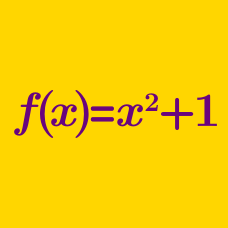Algebra

Composition of Functions

Given two functions:

$f(x)=x+3$

$g(x)=\dfrac{x}{2}$

Find the value of $g(f(5))$.

For the two functions $f(x)=x^2+5x, \, g(x)=x+22,$ what is the value of $(g \circ f)(9)?$

Details and assumptions

$(g \circ f)(x)$ is the composition of $g$ and $f$, defined by $(g \circ f)(x)= g(f(x))$.

Let $f$ be a function such that $(f \circ f)(x) = x^2$

What is the value of $f(f(f(f(f(f(2))))))?$

Consider the two functions $f(x)=6x-5, g(x)=\begin{cases} -x+4 & \text{ if } x \geq 1, \\ 17 & \text{ if } x<1. \end{cases}$ What is the value of $(f \circ g)(2)+(g \circ f)(0)$?

Details and assumptions

$(g \circ f)(x)$ is the composition of $g$ and $f$, defined by $(g \circ f)(x)= g(f(x))$.

$f: \mathbb{R} \rightarrow \mathbb{R}$ is a function satisfying $f( 2x+1) = 4x^2 + 4x$. What is the value of $f(16)$?

×